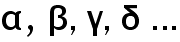Home Math Calculators Math  Flashcards Math Games Math  Homework Math Lessons Math Terms Math Worksheets Math Tools
You Are In Math Glossary of Terms

# Beta

Beta (ß) is a Greek alphabet. In Mathematics, it is used as a mathematical symbol. It is also used in other science subjects.

Example :are similar to a, b, c, d….. in English alphabet.